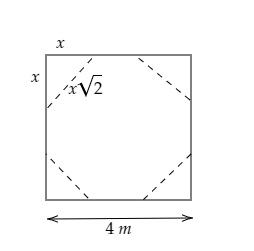# A square, whose side is 4 metres, has its corners cut away so as to form an octagon with all sides equal. Find the length of each side of the octagon (in metres).

Given:

A square, whose side is 4 metres, has its corners cut away so as to form an octagon with all sides equal.

To do:

We have to find the length of each side of the octagon.

Solution:

Let the length of each corner that is cut off be $2x\ m$.

This implies,

The length of the octagon formed $=(4-x-x)\ m=(4-2x)\ m$.From the figure,

We can observe that, the length of the side of the octagon$=\sqrt{x^2+x^2}=x\sqrt{2}$.

Therefore,

$4-2x=x\sqrt2$

$4=x\sqrt2+2x$

$4=x(\sqrt2+2)$

$x=\frac{4}{2+\sqrt2}$

$x=\frac{4}{3.4}$

$x=1.17$

Length of the side of the octagon $=1.17\times1.41=1.65\ m$

The length of each side of the octagon is $1.65\ m$.

Updated on: 10-Oct-2022

155 Views